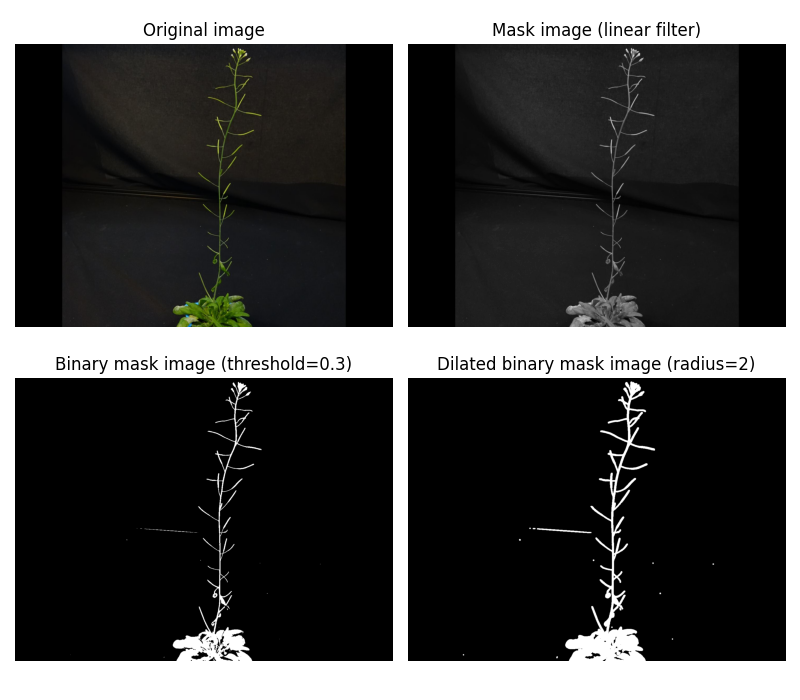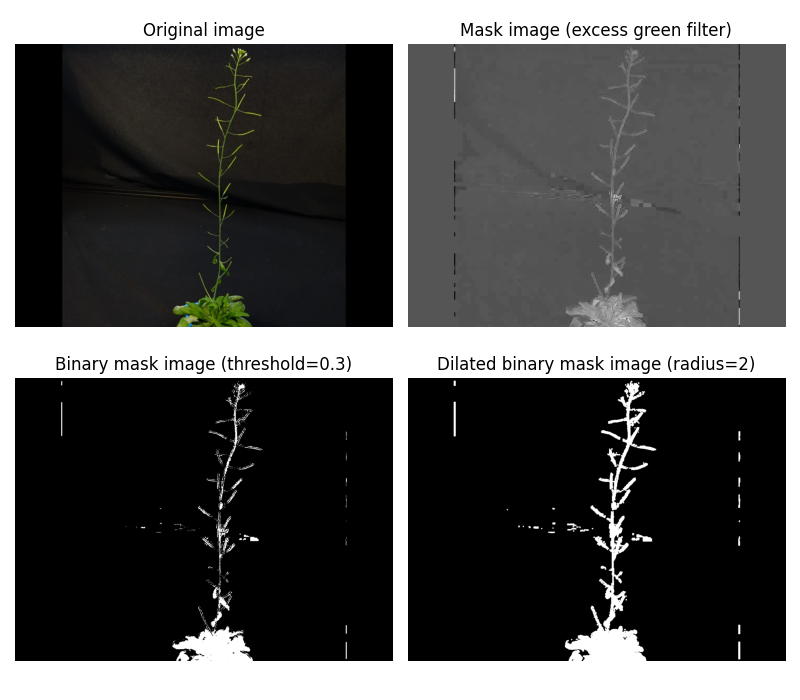Here we show the effects of the type of filter to use in the Mask task.

## Method linearLinkThe image have been generated with the following python code:

import numpy as np
import matplotlib.pyplot as plt
from skimage.exposure import rescale_intensity
from plant3dvision import test_db_path
from plant3dvision.proc2d import linear, dilation
path = test_db_path()
im = np.asarray(im, dtype=float)  # transform the uint8 RGB image into a float RGB numpy array
im = rescale_intensity(im, out_range=(0., 1.))  # rescale to [0., 1.]
threshold = 0.4
filter = linear(im, [0., 1., 0.])  # apply linear filter
mask = filter > threshold  # convert to binary mask using threshold
# Plot the original image, filtered image and binary mask
fig, ax = plt.subplots(1, 3)
ax.imshow(im)
ax.set_title("Original image")
ax.imshow(filter)
ax.set_title("Filtered image")


Important

Pay attention to the values used for threshold and dilation.

## Method excess_greenLinkThe image have been generated with the following python code:

import numpy as np
import matplotlib.pyplot as plt
from skimage.exposure import rescale_intensity
from plant3dvision import test_db_path
from plant3dvision.proc2d import excess_green, dilation
path = test_db_path()
im = np.asarray(im, dtype=float)  # transform the uint8 RGB image into a float RGB numpy array
im = rescale_intensity(im, out_range=(0., 1.))  # rescale to [0., 1.]
threshold = 0.4
filter = excess_green(im)  # apply excess_green filter
mask = filter > threshold  # convert to binary mask using threshold

Pay attention to the values used for threshold and dilation.